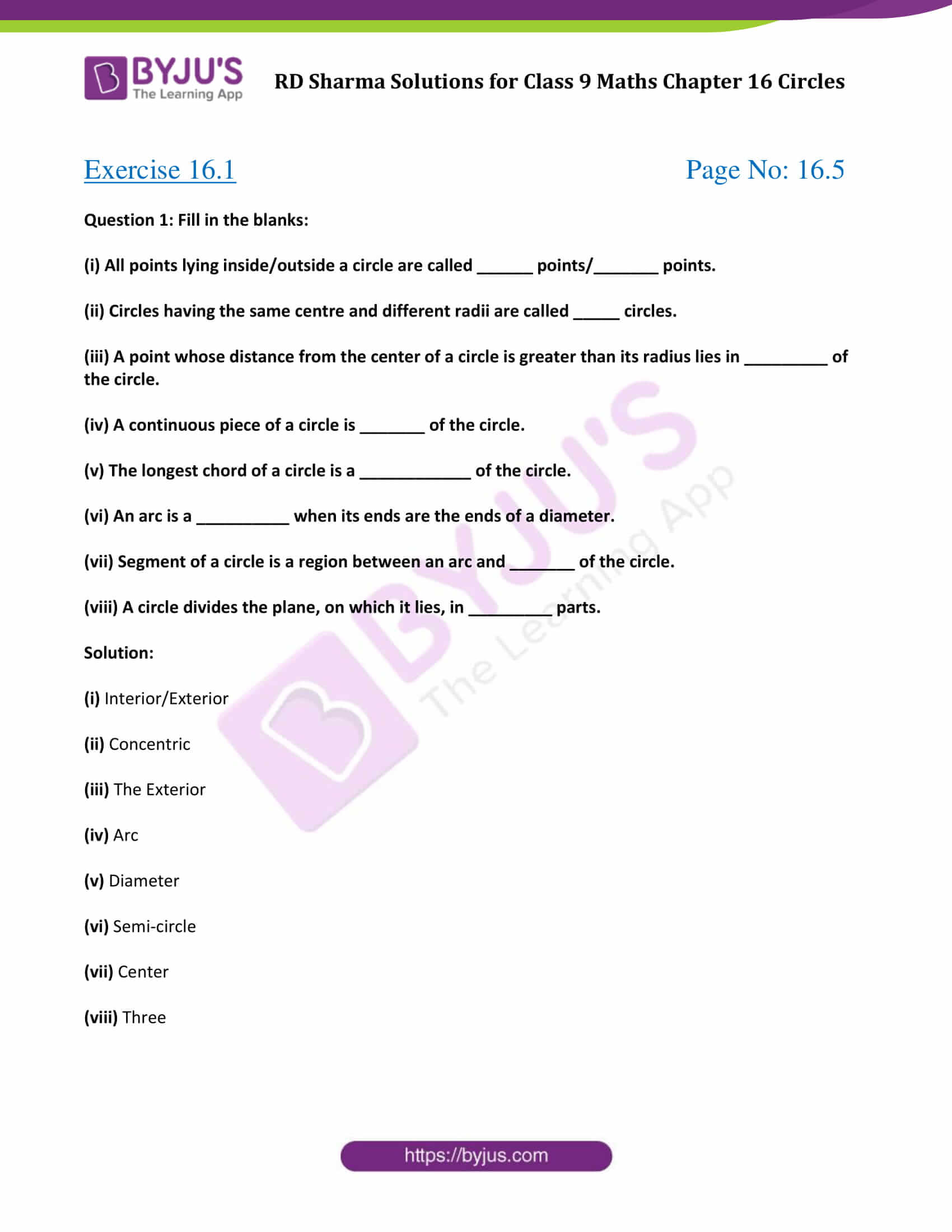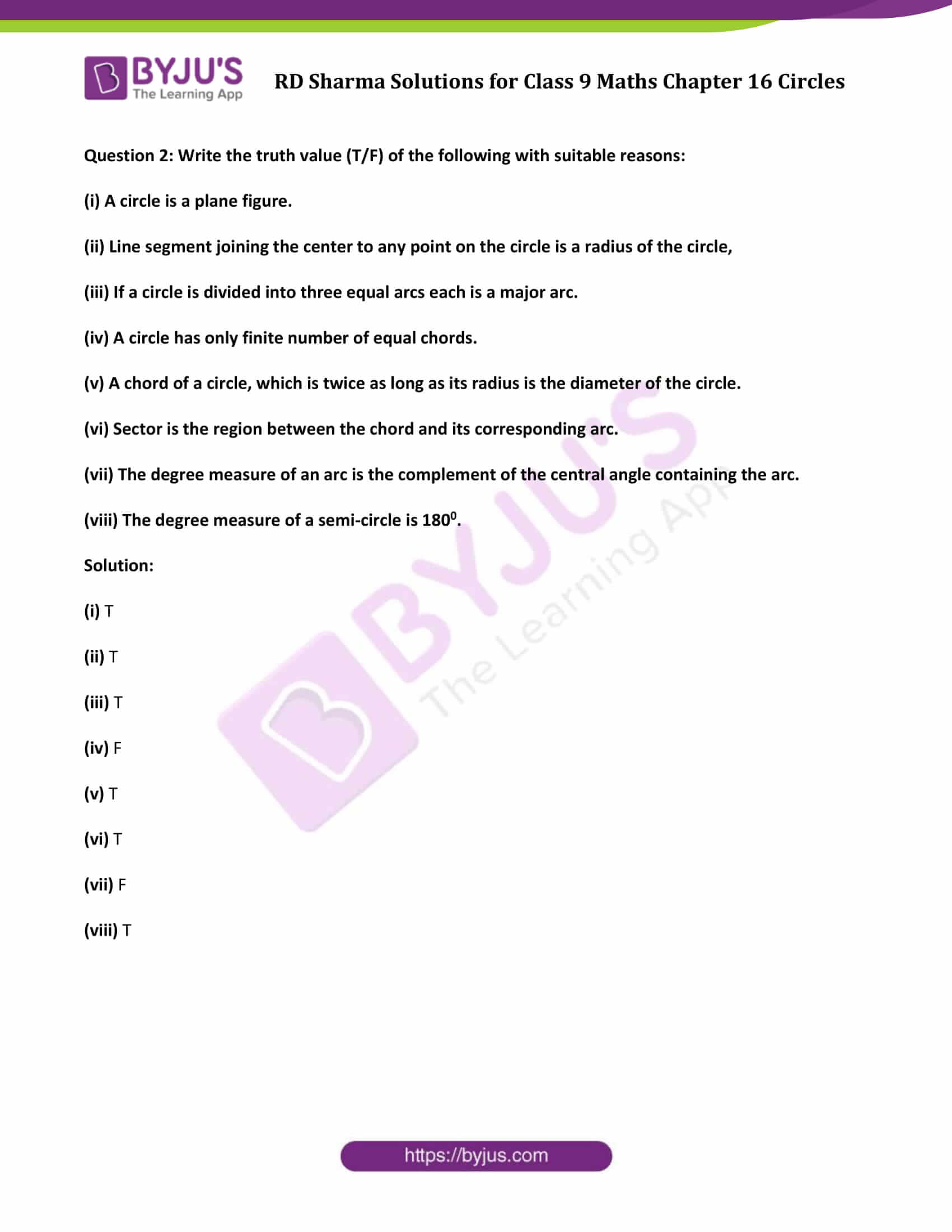# RD Sharma Solutions for Class 9 Maths Chapter 16 Circles Exercise 16.1

Get free RD Sharma Solutions for Class 9 Maths Chapter 16 exercise 16.1 – Circles here. This study material is based on the latest syllabus of CBSE board syllabus. Students can access all the RD Sharma class 9 solutions in the exact sequence as in the textbook. Click on the link below to get your pdf now.

## Download PDF of RD Sharma Solutions for Class 9 Maths Chapter 16 Circles Exercise 16.1### Access Answers to Maths RD Sharma Solutions for Class 9 Chapter 16 Circles Exercise 16.1 Page number 16.5

Question 1: Fill in the blanks:

(i) All points lying inside/outside a circle are called ______ points/_______ points.

(ii) Circles having the same centre and different radii are called _____ circles.

(iii) A point whose distance from the center of a circle is greater than its radius lies in _________ of the circle.

(iv) A continuous piece of a circle is _______ of the circle.

(v) The longest chord of a circle is a ____________ of the circle.

(vi) An arc is a __________ when its ends are the ends of a diameter.

(vii) Segment of a circle is a region between an arc and _______ of the circle.

(viii) A circle divides the plane, on which it lies, in _________ parts.

Solution:

(i) Interior/Exterior

(ii) Concentric

(iii) The Exterior

(iv) Arc

(v) Diameter

(vi) Semi-circle

(vii) Center

(viii) Three

Question 2: Write the truth value (T/F) of the following with suitable reasons:

(i) A circle is a plane figure.

(ii) Line segment joining the center to any point on the circle is a radius of the circle,

(iii) If a circle is divided into three equal arcs each is a major arc.

(iv) A circle has only finite number of equal chords.

(v) A chord of a circle, which is twice as long as its radius is the diameter of the circle.

(vi) Sector is the region between the chord and its corresponding arc.

(vii) The degree measure of an arc is the complement of the central angle containing the arc.

(viii) The degree measure of a semi-circle is 1800.

Solution:

(i) T

(ii) T

(iii) T

(iv) F

(v) T

(vi) T

(vii) F

(viii) T

## RD Sharma Solutions for Class 9 Maths Chapter 16 Circles Exercise 16.1

RD Sharma Solutions Class 9 Maths Chapter 16 Circles Exercise 16.1 is based on the following topics and subtopics:

• Position of a point with respect to a circle
• Circular Disc
• Concentric Circles
• Arc of a circle
• Length of an arc
• Central Angle
• Minor Arc
• Major Arc
• Degree measure of an arc
• Chord and segment of a circle

-Diameter

– Semi-circle

-Segment of a circle

– Alternate segment of a circle